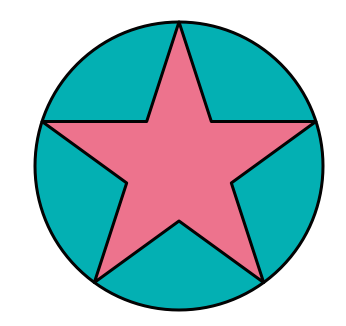# The Star Medallion

Geometry Level 3A pentagram is inscribed inside a circle with radius $r$ as shown in the picture above. The formula that puts the area of the pentagram $S$ in terms of the circle's radius $r$ can be expressed as follows:

$\large S = \dfrac{a \sqrt{10a-b\sqrt a}}c r^2 \; ,$

where $a,b$ and $c$ are positive integers with $a$ square-free and $a,c$ coprime.

Find the value of $a+b+c$.

×